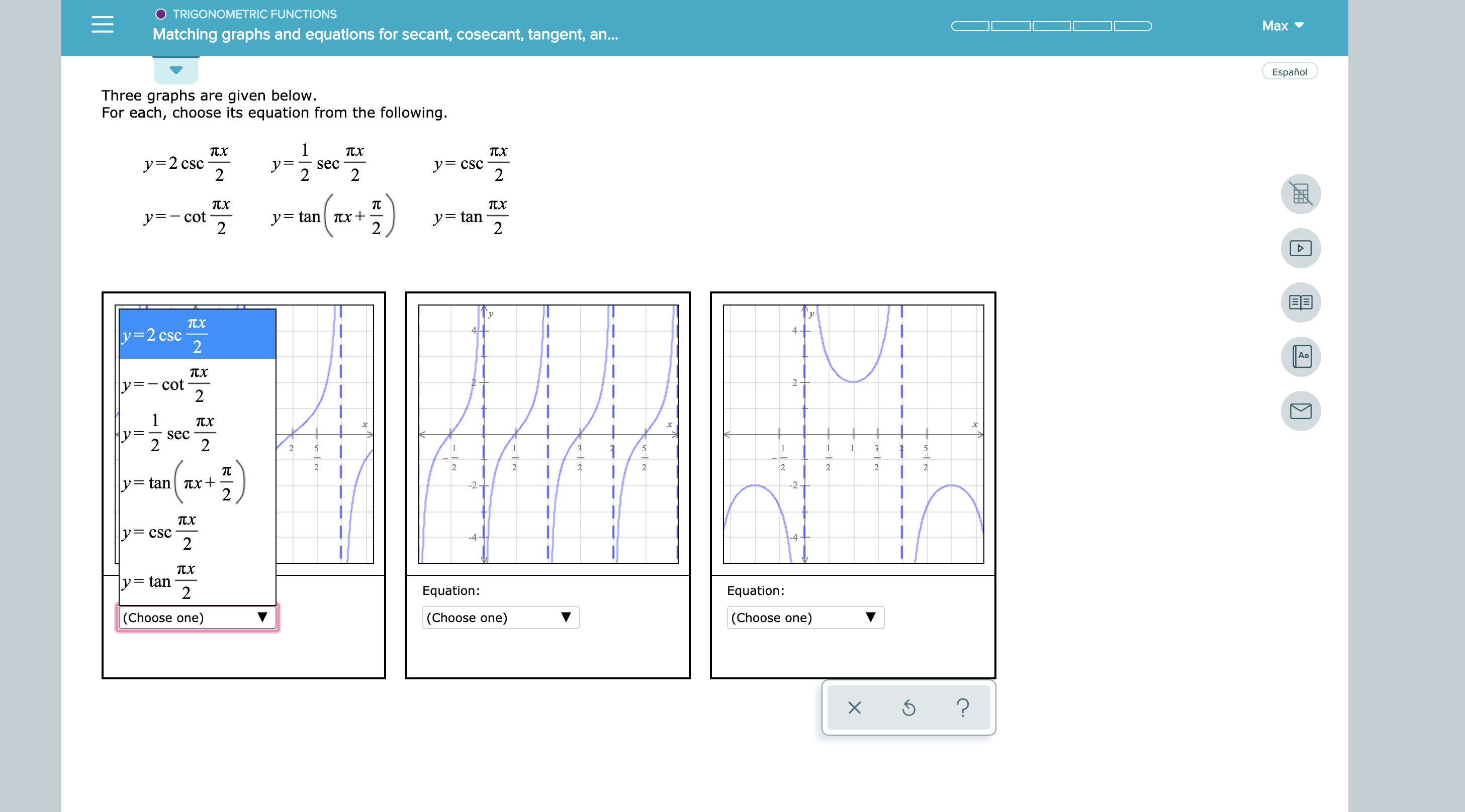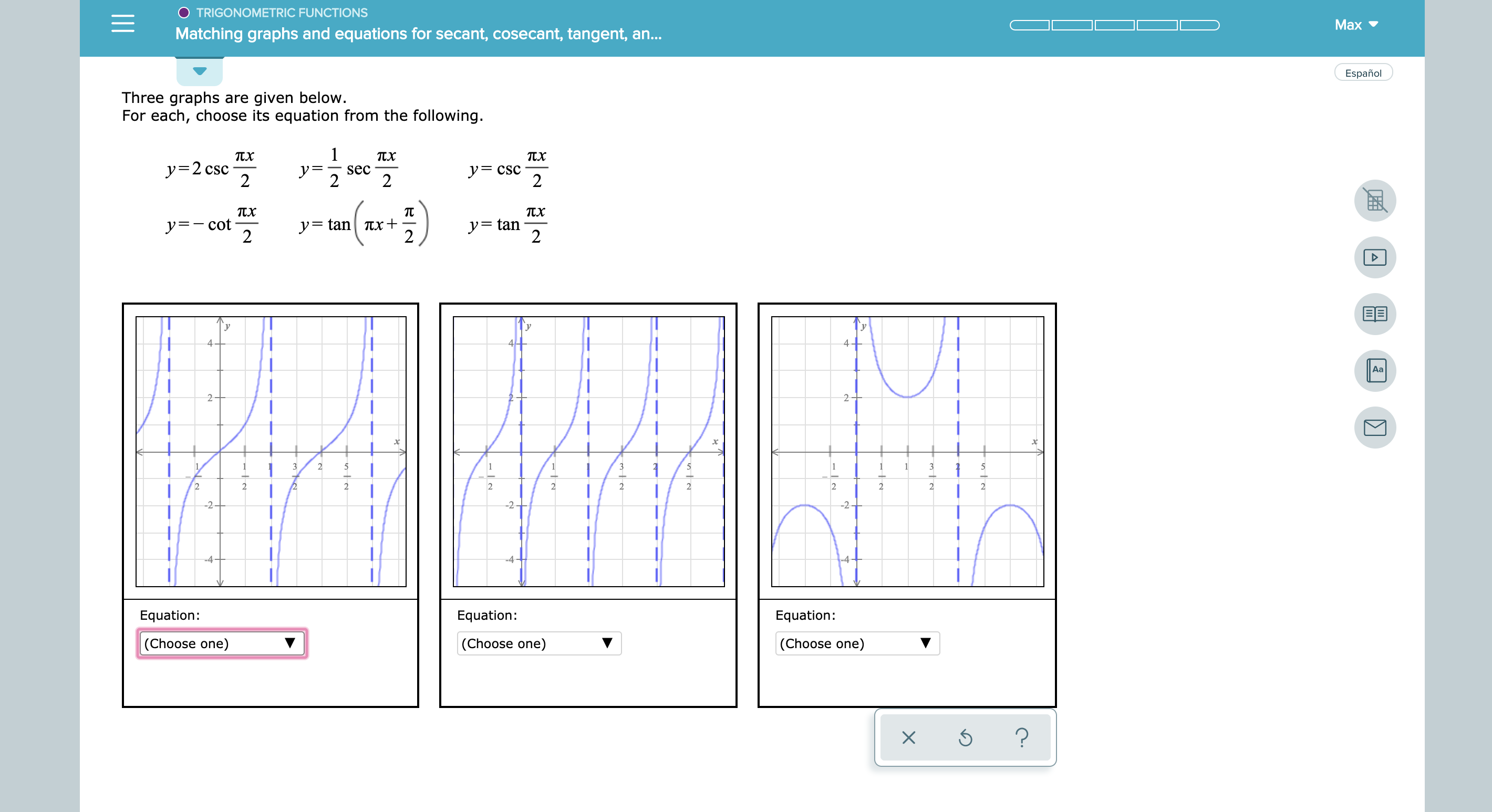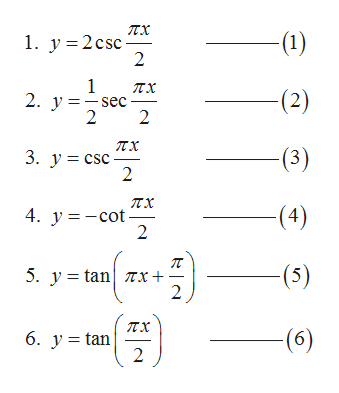# O TRIGONOMETRIC FUNCTIONSMaxMatching graphs and equations for secant, cosecant, tangent, an...EspañolThree graphs are given below.For each, choose its equation from the following.1TXTxy=2 csc2y= cSC2sec2y=tan2ytan Tx+2ア=ー cot2TX4y=2 cscAaTXcot2-1sec212111322222222y=tanTx+2-22yCSC2-4y= tan2Equation:Equation:|(Choose one)(Choose one)(Choose one)? TRIGONOMETRIC FUNCTIONSMaxMatching graphs and equations for secant, cosecant, tangent, an...EspañolThree graphs are given below.For each, choose its equation from the following.1TXsec2TXTXy 2 csc2yCSC22TCytan 7x+2y cot2y=tan2EEAa2-2-23513222222222222-2-2-2-4--4Equation:Equation:Equation:(Choose one)(Choose one)(Choose one)?

Question
9 views

see attachmenthelp_outlineImage TranscriptioncloseO TRIGONOMETRIC FUNCTIONS Max Matching graphs and equations for secant, cosecant, tangent, an... Español Three graphs are given below. For each, choose its equation from the following. 1 TX Tx y=2 csc 2 y= cSC 2 sec 2 y=tan 2 ytan Tx+ 2 ア=ー cot 2 TX 4 y=2 csc Aa TX cot 2- 1 sec 2 1 2 1 1 1 3 2 2 2 2 2 2 2 2 y=tanTx+ 2 -2 2 yCSC 2 -4 y= tan 2 Equation: Equation: |(Choose one) (Choose one) (Choose one) ? fullscreenhelp_outlineImage TranscriptioncloseTRIGONOMETRIC FUNCTIONS Max Matching graphs and equations for secant, cosecant, tangent, an... Español Three graphs are given below. For each, choose its equation from the following. 1 TX sec 2 TX TX y 2 csc 2 yCSC 2 2 TC ytan 7x+ 2 y cot 2 y=tan 2 EE Aa 2- 2- 2 3 5 1 3 2 2 2 2 2 2 2 2 2 2 2 2 -2- 2 -2 -4- -4 Equation: Equation: Equation: (Choose one) (Choose one) (Choose one) ? fullscreen
check_circle

Step 1

The given equations are as following:help_outlineImage Transcriptioncloseпх 1. у%3D2csc 2 (1) 1 пх 2. у %3D— sec 2 -(2) 2 пх 3. у%3D csc 2 -(3) пх 4. у%3D— сot. 2 (4) п 5. у %3 tan| zх + 2 (5) пх 6. у %3Dtan 2 (6) fullscreen
Step 2

It can be clearly be seen that the first two graphs are the graph of either tan or cot trigonometric functions. So, these two graphs can only be represented by equation 4, 5 or 6 only.

Step 3

In the first graph,
when x = 0 the y becomes 0 and
when x = 1 the y becomes infinity
which can only possible in...

### Want to see the full answer?

See Solution

#### Want to see this answer and more?

Solutions are written by subject experts who are available 24/7. Questions are typically answered within 1 hour.*

See Solution
*Response times may vary by subject and question.
Tagged in

### Functions